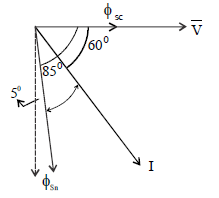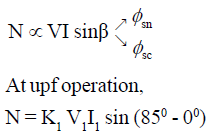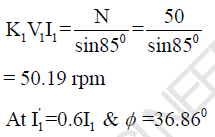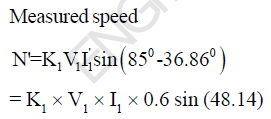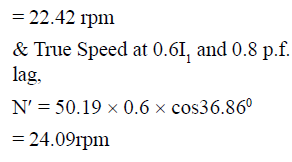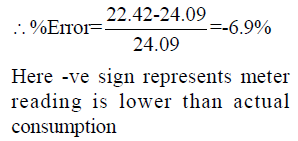Courses

# Measurement

## 25 Questions MCQ Test GATE Electrical Engineering (EE) 2022 Mock Test Series | Measurement

Description
This mock test of Measurement for GATE helps you for every GATE entrance exam. This contains 25 Multiple Choice Questions for GATE Measurement (mcq) to study with solutions a complete question bank. The solved questions answers in this Measurement quiz give you a good mix of easy questions and tough questions. GATE students definitely take this Measurement exercise for a better result in the exam. You can find other Measurement extra questions, long questions & short questions for GATE on EduRev as well by searching above.
QUESTION: 1

Solution:
QUESTION: 2

Solution:
QUESTION: 3

### The Eddy current damping is not used in electrodynamometer type instrument as

Solution:
QUESTION: 4

Manganin is preferred to constantan as a shunt wire in Ammeter in d.c. application, it is to

Solution:
QUESTION: 5

The limiting values of voltage, current and power factor of 1-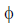induction motor is given as 200V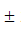5%, 10A2% and 0.810%. The limiting error of reactive power input is

Solution: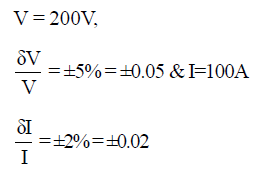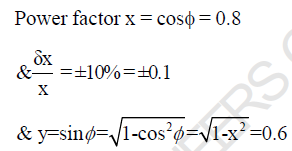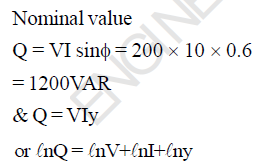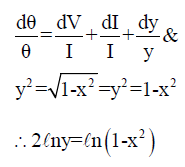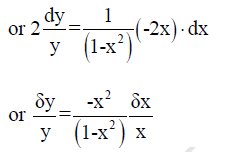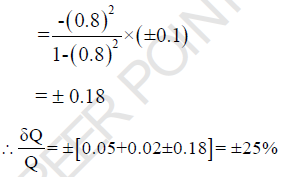QUESTION: 6

The current through the current coil of wattmeter is 2 + 3 cos 2(t - 300) + 5cos(4t - 600) and voltage across
its pressure coil is 1 + 4 sin(2t - 300) + 3 sin (6t - 100). The reading of wattmeter will be

Solution: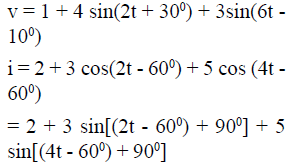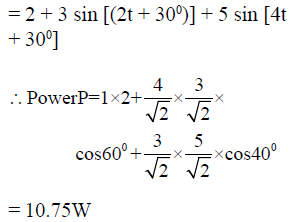QUESTION: 7

The inductance of a certain moving iron ammeter is given as L = 5 + 2θ - θ2μH where deflection θ is in
radians from zero position. If spring constant is 20 μNm/rad. The deflection for a current of 1A is

Solution: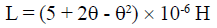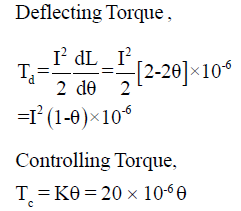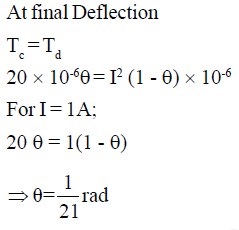QUESTION: 8

Two 50V full scale PMMC type D.C. Voltmeters having sensitivities of 5K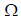/V and 10K/V are
connected in series. The sensitivity of the series combination is

Solution: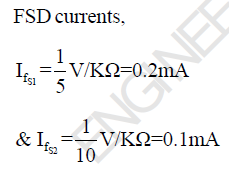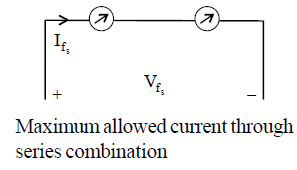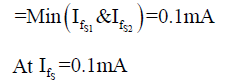First meter shows half of FSD i.e.
25V and second meter shows FDS
i.e. SoV.
So voltage that can be measured
using combination = 75V.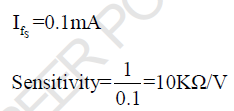QUESTION: 9

A 10A D.C. Ammeter has a resistance of 0.1is to be extended to 50A, the required shunt wire is

Solution: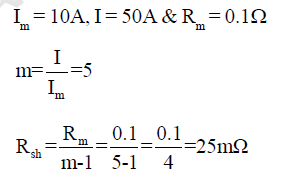QUESTION: 10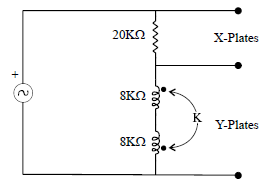From the above circuit, the voltages are applied to X and Y plates, the value of coefficient of coupling to
observe a circle on CRO screen, (X and Y plates have equal V/div.)

Solution: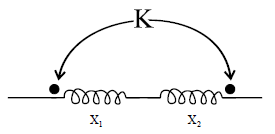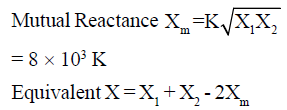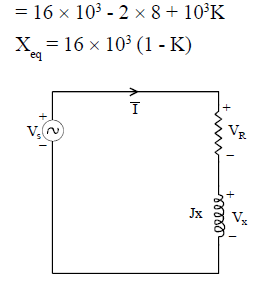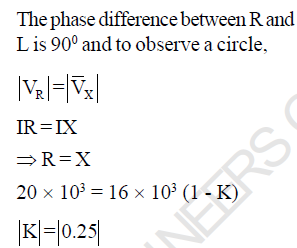QUESTION: 11

A wattmeter reads P when its current coil is connected in the phase A and its PC is between phase
B & C of symmetrical 3-ABC system supplying a balanced Yconnected 0.6 pf capacitive load,
what will be the reading of wattmeter if CC is reversed as well as phase sequence is reversed by
interchanging phases B & C

Solution: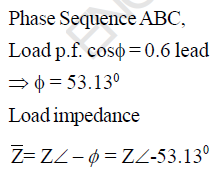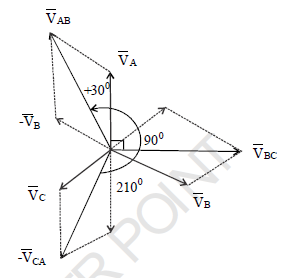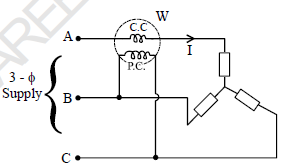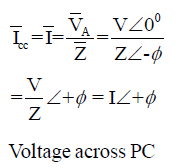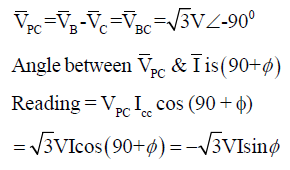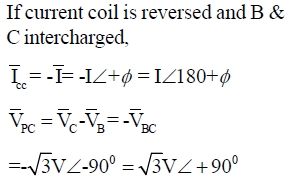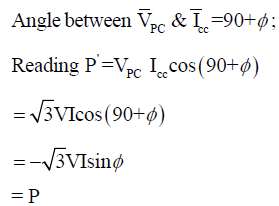QUESTION: 12

In the wheatstone Bridge the V0 is (approximately)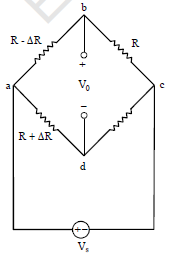Solution: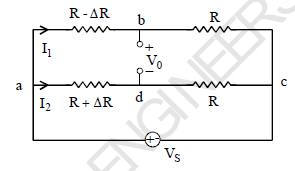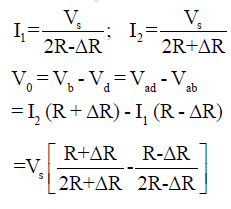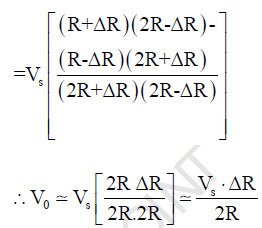QUESTION: 13

A potentiometric transducer supplied by 10V d.c. and having total resistance 10K is used to
measure the linear displacement. At 60% of total travel to obtain the accuracy better than 98% for
measurement, the voltmeter should have a resistance atleast (approximately)

Solution: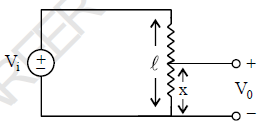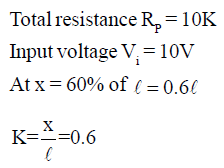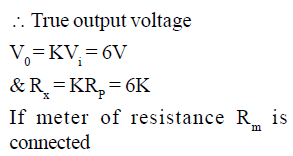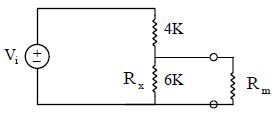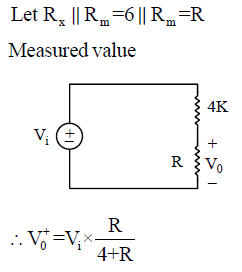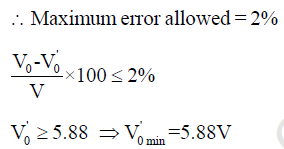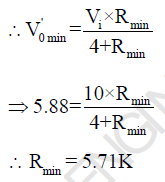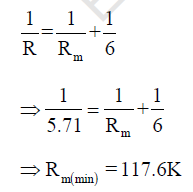QUESTION: 14

In the measurment of power of balanced load by two wattmeter method in a 3-circuit. The readings of the wattmeters are 4kW and 2 kW respectively, the later is taken after reversing the connections of current coil. The power factor and reactive power of the load is

Solution: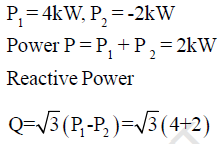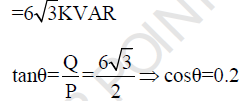QUESTION: 15

A strain Gauge with Gauge factor 4 is bonded to a steel beam is subjected to a stress of 5 x 105 kN/
m2. The modulus of elasticity of steel of 20 GN/m2, the change in the resistance of guage is

Solution: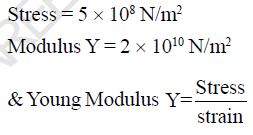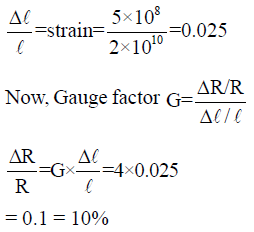QUESTION: 16

An electrodynamometer type wattmeter is provided with a capacitor across the resistance in its Potential coil. It is required mainly when

Solution:

In Low power factor ED type
wattmeter Potential coil inductance
is compensated by capacitor across
the resistance of PC.

QUESTION: 17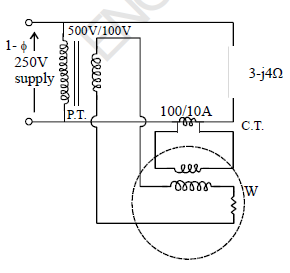In the circuit shown the reading of wattmeter is

Solution: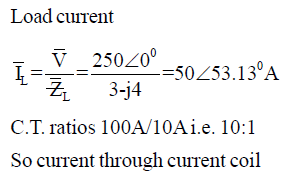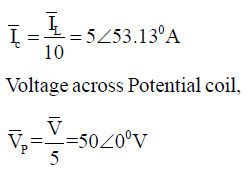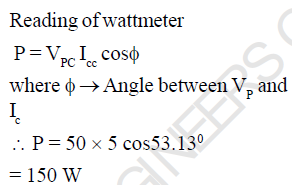QUESTION: 18

A 10KV, 50Hz supply is fed to Bridge rectifier type (using ideal diodes) EVM through a capacitor.
The 1A PMMC, of negligible resistance, is provided in series with 6Kresistance. The value of
capacitor to obtain 90% of full obtain deflection in PMMC is

Solution: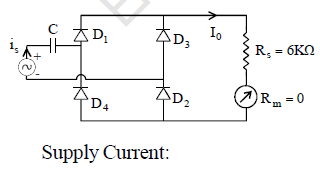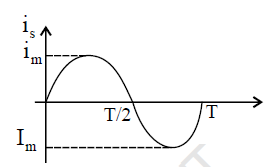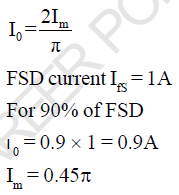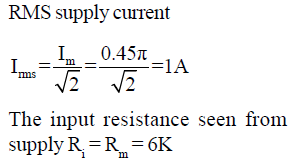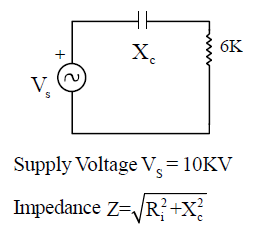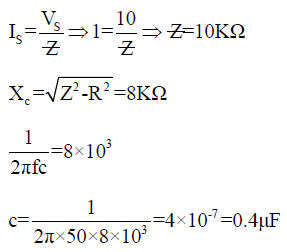QUESTION: 19

In a 100V PMMC type voltmeter, where 40V is measured, the pointer goes upto the 40.6 before finally
settling at 40V. If due to the increment in temperature, stiffness of spring gets reduced by 20%. The
maximum swing of pointer when measured 80% of FSD, is

Solution: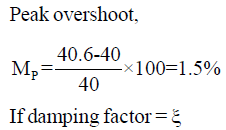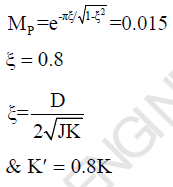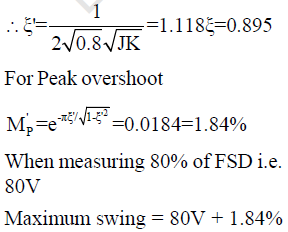of 80V = 81.47V

QUESTION: 20

A resistance is measured by the voltmeter-ammeter method employing d.c. excitation and a voltmeter of very high resistance connected directly across the unknown resistance. If the voltmeter and ammeter readings are subject to maximum possible errors of2.4% and1% respectively,
than the magnitude of the maximum possible percentage error in the value of resistance deduced
from the measurement is nearly

Solution: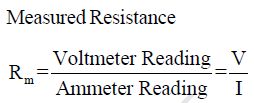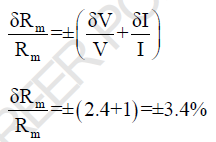QUESTION: 21

In the Wheatstone Bridge shown in figure.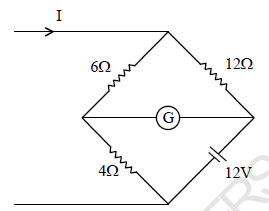Current I in the shown figure is,

Solution:

Given WSB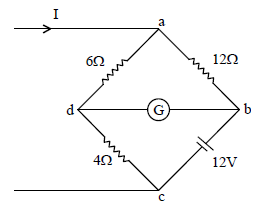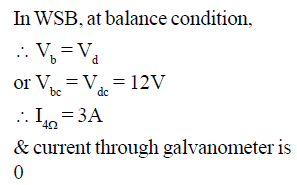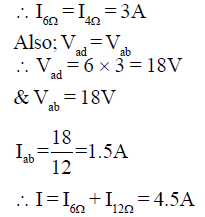QUESTION: 22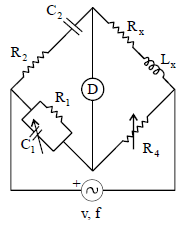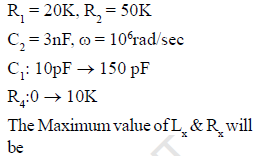Solution: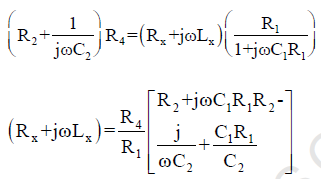Therefore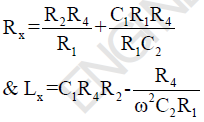For Rx max; R4 & C1 is maximum
& for Lx max; C1 max & R4 is
maximum

.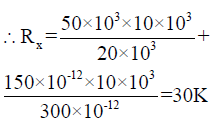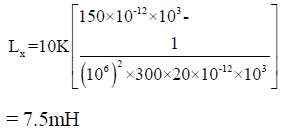QUESTION: 23

The current coil and potential coil of a dynamometer type wattmeter were accidently interchanged while
connecting, After energizing the circuit, it was observed that the wattmeter did not show the reading.
This could be due to the

Solution:

As the resistance of current coil is
low if it is connected across high
voltage , the maximum current is
flowing through it and a large
voltage drop takes place due to
which it gets damaged.

QUESTION: 24

In the circuit shown in the given figure, the wattmeter Reading will be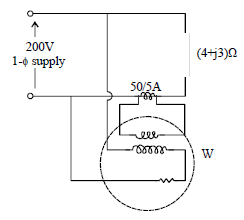Solution: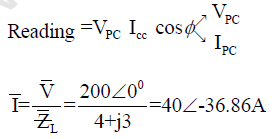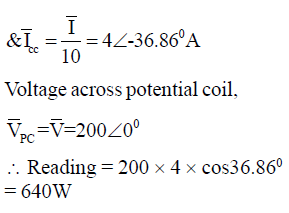*Answer can only contain numeric values
QUESTION: 25

In an energy meter, the current and flux adjustment of series magnet are in phase while there is an angular
departure of 50 from quadrature between voltage and shunt magnetic flux. The speed of disc at
full load and unity power factor is 50 rpm. The error of the disc at 60% of full load and 0.8 power
factor lag is_____

Solution: